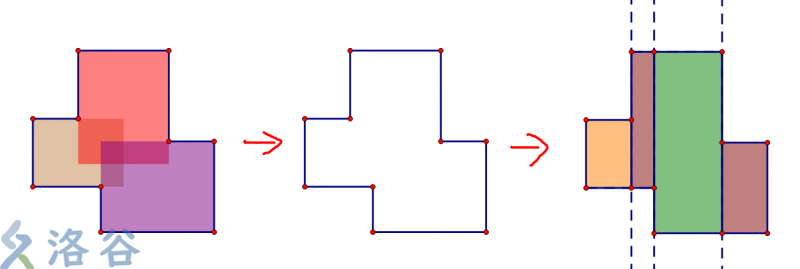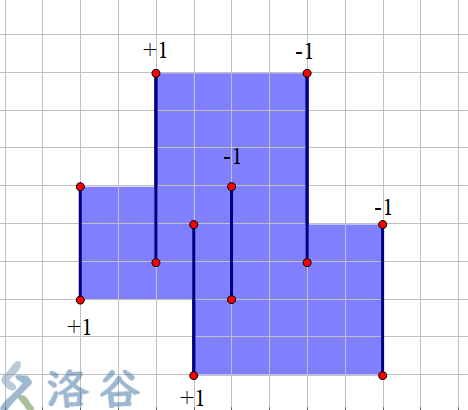# categories/刷题记录/共658篇文章zc
2019-12-21 19:47:00zc
2019-12-21 19:47:00zc
2019-12-21 19:47:00

f_i表示[1,i]最多变成多少个

f_i=\max_{s_i=s_j}(f[j-1]+s_j\times (\sum_{i=j}^i[s_i=s_j])^2)zc
2019-12-21 19:47:00

（代码很短）zc
2019-12-21 19:47:00zc
2019-12-21 19:47:00

i,i^2,i^3...,i^k都会被去掉

ans = \sum_{i=1}^n \frac 1 {d_i}

n = p_1^{k_1}p_2^{k_2}p_3^{k_3}p_4^{k_4}...zc
2019-12-21 19:47:00

## 解法

s_i=\sum_{j=1}^m a_{ij}

\because k=\frac {tot}2

f(i,k)表示总共选了i次(也就是选到第i行)权值为k的方案数

f(i,k) += f(i-1,k)*(s_i-a_{ij})(当前行不选目标列

f(i,k+1) += f(i-1,k)(当前行完全不选

f(i,k+2) += f(i-1,k)\times a_{ij}(当前行选中目标列

ans = \prod_{i=1}^n (s_i+1) - 1 - \sum_{i=n+1}^{2n} f(n,i)zc
2019-12-21 19:47:00

f_i为前i个任务的费用,t_i为前i个任务时间的总和,w_i为前i个任务费用的总和

 f_i=\min_{j=0}^{i-1}(f_j+s\times(w_n-w_j)+t_i\times(w_i-w_j)) 

 f_j+s\times(w_n-w_j)+t_i\times(w_i-w_j) \le f_k+s\times(w_n-w_k)+t_i\times(w_i-w_k) \\ t_i(w_k-w_j)\le f_k-f_j+s(w_j-w_k) \\ t_i\le \frac{f_k-f_j+s(w_j-w_k)}{w_k-w_j}zc
2019-12-21 19:47:00

(a,b,c) , (b,a,c)这样的是不符合的,减去其中一个

...

https://www.luogu.com.cn/blog/lingchi/solution-cf1008dzc
2019-12-21 19:47:00

Search
search